#《利用Python进行数据分析·第2版》第4章 NumPy基础：数组和矢量计算

0
0
01. 云栖社区>
2. 博客>
3. 正文

## 《利用Python进行数据分析·第2版》第4章 NumPy基础：数组和矢量计算

seancheney 2017-11-09 17:52:00 浏览805

NumPy（Numerical Python的简称）是Python数值计算最重要的基础包。大多数提供科学计算的包都是用NumPy的数组作为构建基础。

NumPy的部分功能如下：

• ndarray，一个具有矢量算术运算和复杂广播能力的快速且节省空间的多维数组。
• 用于对整组数据进行快速运算的标准数学函数（无需编写循环）。
• 用于读写磁盘数据的工具以及用于操作内存映射文件的工具。
• 线性代数、随机数生成以及傅里叶变换功能。
• 用于集成由C、C++、Fortran等语言编写的代码的A C API。

NumPy本身并没有提供多么高级的数据分析功能，理解NumPy数组以及面向数组的计算将有助于你更加高效地使用诸如pandas之类的工具。因为NumPy是一个很大的题目，我会在附录A中介绍更多NumPy高级功能，比如广播。

• 用于数据整理和清理、子集构造和过滤、转换等快速的矢量化数组运算。
• 常用的数组算法，如排序、唯一化、集合运算等。
• 高效的描述统计和数据聚合/摘要运算。
• 用于异构数据集的合并/连接运算的数据对齐和关系型数据运算。
• 将条件逻辑表述为数组表达式（而不是带有if-elif-else分支的循环）。
• 数据的分组运算（聚合、转换、函数应用等）。。

NumPy之于数值计算特别重要的原因之一，是因为它可以高效处理大数组的数据。这是因为：

• NumPy是在一个连续的内存块中存储数据，独立于其他Python内置对象。NumPy的C语言编写的算法库可以操作内存，而不必进行类型检查或其它前期工作。比起Python的内置序列，NumPy数组使用的内存更少。
• NumPy可以在整个数组上执行复杂的计算，而不需要Python的for循环。

In : import numpy as np

In : my_arr = np.arange(1000000)

In : my_list = list(range(1000000))


In : %time for _ in range(10): my_arr2 = my_arr * 2
CPU times: user 20 ms, sys: 50 ms, total: 70 ms
Wall time: 72.4 ms

In : %time for _ in range(10): my_list2 = [x * 2 for x in my_list]
CPU times: user 760 ms, sys: 290 ms, total: 1.05 s
Wall time: 1.05 s


# 4.1 NumPy的ndarray：一种多维数组对象

NumPy最重要的一个特点就是其N维数组对象（即ndarray），该对象是一个快速而灵活的大数据集容器。你可以利用这种数组对整块数据执行一些数学运算，其语法跟标量元素之间的运算一样。

In : import numpy as np

# Generate some random data
In : data = np.random.randn(2, 3)

In : data
Out:
array([[-0.2047,  0.4789, -0.5194],
[-0.5557,  1.9658,  1.3934]])


In : data * 10
Out:
array([[ -2.0471,   4.7894,  -5.1944],
[ -5.5573,  19.6578,  13.9341]])

In : data + data
Out:
array([[-0.4094,  0.9579, -1.0389],
[-1.1115,  3.9316,  2.7868]])


ndarray是一个通用的同构数据多维容器，也就是说，其中的所有元素必须是相同类型的。每个数组都有一个shape（一个表示各维度大小的元组）和一个dtype（一个用于说明数组数据类型的对象）：

In : data.shape
Out: (2, 3)

In : data.dtype
Out: dtype('float64')


## 创建ndarray

In : data1 = [6, 7.5, 8, 0, 1]

In : arr1 = np.array(data1)

In : arr1
Out: array([ 6. ,  7.5,  8. ,  0. ,  1. ])


In : data2 = [[1, 2, 3, 4], [5, 6, 7, 8]]

In : arr2 = np.array(data2)

In : arr2
Out:
array([[1, 2, 3, 4],
[5, 6, 7, 8]])


In : arr2.ndim
Out: 2

In : arr2.shape
Out: (2, 4)


In : arr1.dtype
Out: dtype('float64')

In : arr2.dtype
Out: dtype('int64')


In : np.zeros(10)
Out: array([ 0.,  0.,  0.,  0.,  0.,  0.,  0.,  0.,  0.,  0.])

In : np.zeros((3, 6))
Out:
array([[ 0.,  0.,  0.,  0.,  0.,  0.],
[ 0.,  0.,  0.,  0.,  0.,  0.],
[ 0.,  0.,  0.,  0.,  0.,  0.]])

In : np.empty((2, 3, 2))
Out:
array([[[ 0.,  0.],
[ 0.,  0.],
[ 0.,  0.]],
[[ 0.,  0.],
[ 0.,  0.],
[ 0.,  0.]]])


arange是Python内置函数range的数组版：

In : np.arange(15)
Out: array([ 0,  1,  2,  3,  4,  5,  6,  7,  8,  9, 10, 11, 12, 13, 14])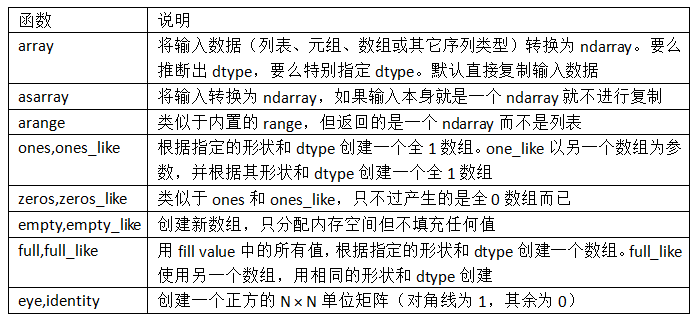## ndarray的数据类型

dtype（数据类型）是一个特殊的对象，它含有ndarray将一块内存解释为特定数据类型所需的信息：

In : arr1 = np.array([1, 2, 3], dtype=np.float64)

In : arr2 = np.array([1, 2, 3], dtype=np.int32)

In : arr1.dtype
Out: dtype('float64')

In : arr2.dtype
Out: dtype('int32')


dtype是NumPy灵活交互其它系统的源泉之一。多数情况下，它们直接映射到相应的机器表示，这使得“读写磁盘上的二进制数据流”以及“集成低级语言代码（如C、Fortran）”等工作变得更加简单。数值型dtype的命名方式相同：一个类型名（如float或int），后面跟一个用于表示各元素位长的数字。标准的双精度浮点值（即Python中的float对象）需要占用8字节（即64位）。因此，该类型在NumPy中就记作float64。表4-2列出了NumPy所支持的全部数据类型。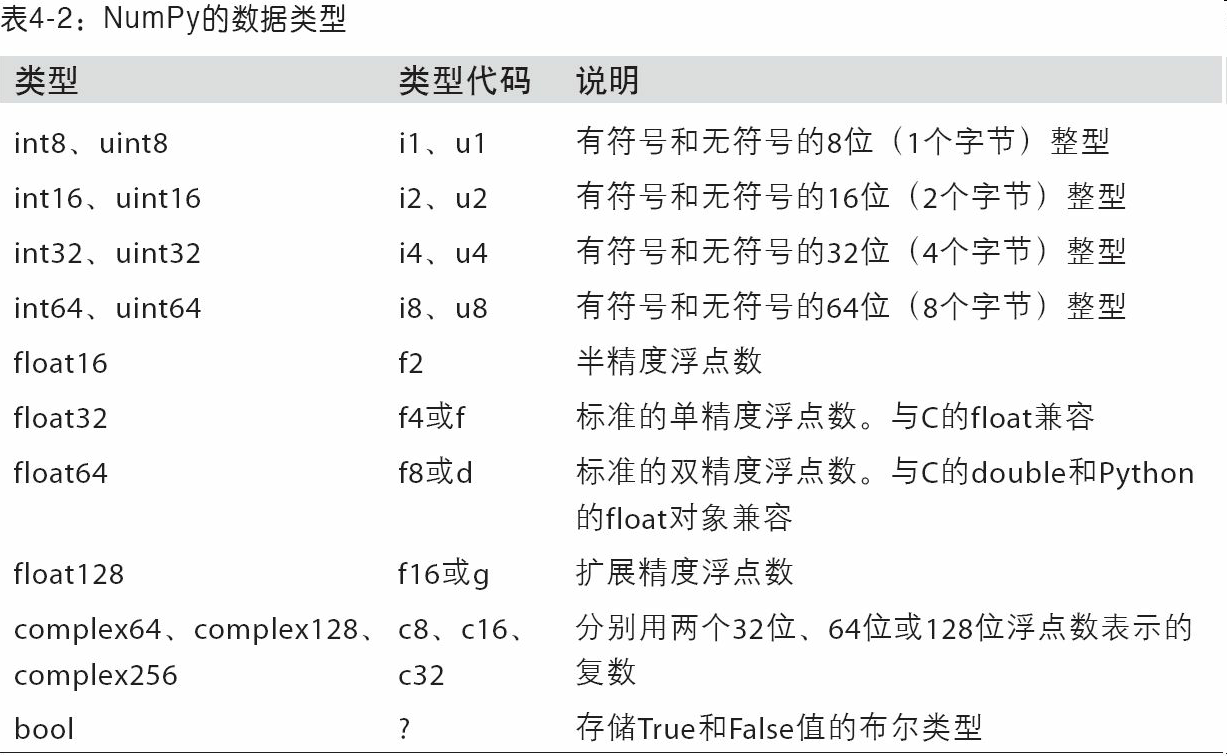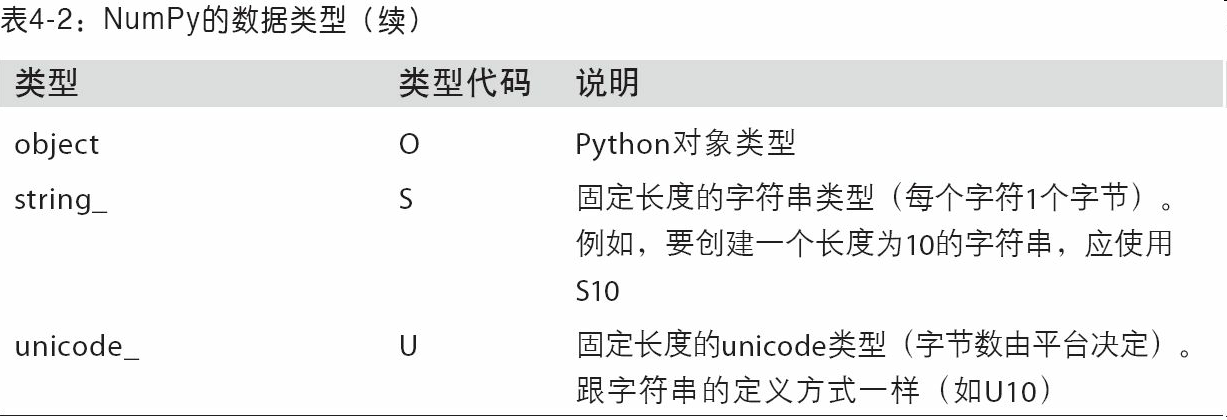In : arr = np.array([1, 2, 3, 4, 5])

In : arr.dtype
Out: dtype('int64')

In : float_arr = arr.astype(np.float64)

In : float_arr.dtype
Out: dtype('float64')


In : arr = np.array([3.7, -1.2, -2.6, 0.5, 12.9, 10.1])

In : arr
Out: array([  3.7,  -1.2,  -2.6,   0.5,  12.9,  10.1])

In : arr.astype(np.int32)
Out: array([ 3, -1, -2,  0, 12, 10], dtype=int32)


In : numeric_strings = np.array(['1.25', '-9.6', '42'], dtype=np.string_)

In : numeric_strings.astype(float)
Out: array([  1.25,  -9.6 ,  42.  ])


In : int_array = np.arange(10)

In : calibers = np.array([.22, .270, .357, .380, .44, .50], dtype=np.float64)

In : int_array.astype(calibers.dtype)
Out: array([ 0.,  1.,  2.,  3.,  4.,  5.,  6.,  7.,  8.,  9.])


In : empty_uint32 = np.empty(8, dtype='u4')

In : empty_uint32
Out:
array([         0, 1075314688,          0, 1075707904,          0,
1075838976,          0, 1072693248], dtype=uint32)


## NumPy数组的运算

In : arr = np.array([[1., 2., 3.], [4., 5., 6.]])

In : arr
Out:
array([[ 1.,  2.,  3.],
[ 4.,  5.,  6.]])

In : arr * arr
Out:
array([[  1.,   4.,   9.],
[ 16.,  25.,  36.]])

In : arr - arr
Out:
array([[ 0.,  0.,  0.],
[ 0.,  0.,  0.]])


In : 1 / arr
Out:
array([[ 1.    ,  0.5   ,  0.3333],
[ 0.25  ,  0.2   ,  0.1667]])

In : arr ** 0.5
Out:
array([[ 1.    ,  1.4142,  1.7321],
[ 2.    ,  2.2361,  2.4495]])


In : arr2 = np.array([[0., 4., 1.], [7., 2., 12.]])

In : arr2
Out:
array([[  0.,   4.,   1.],
[  7.,   2.,  12.]])

In : arr2 > arr
Out:
array([[False,  True, False],
[ True, False,  True]], dtype=bool)


## 基本的索引和切片

NumPy数组的索引是一个内容丰富的主题，因为选取数据子集或单个元素的方式有很多。一维数组很简单。从表面上看，它们跟Python列表的功能差不多：

In : arr = np.arange(10)

In : arr
Out: array([0, 1, 2, 3, 4, 5, 6, 7, 8, 9])

In : arr
Out: 5

In : arr[5:8]
Out: array([5, 6, 7])

In : arr[5:8] = 12

In : arr
Out: array([ 0,  1,  2,  3,  4, 12, 12, 12,  8,  9])


In : arr_slice = arr[5:8]

In : arr_slice
Out: array([12, 12, 12])


In : arr_slice = 12345

In : arr
Out: array([    0,     1,     2,     3,     4,    12, 12345,    12,     8,
9])


In : arr_slice[:] = 64

In : arr
Out: array([ 0,  1,  2,  3,  4, 64, 64, 64,  8,  9])


In : arr2d = np.array([[1, 2, 3], [4, 5, 6], [7, 8, 9]])

In : arr2d
Out: array([7, 8, 9])


In : arr2d
Out: 3

In : arr2d[0, 2]
Out: 3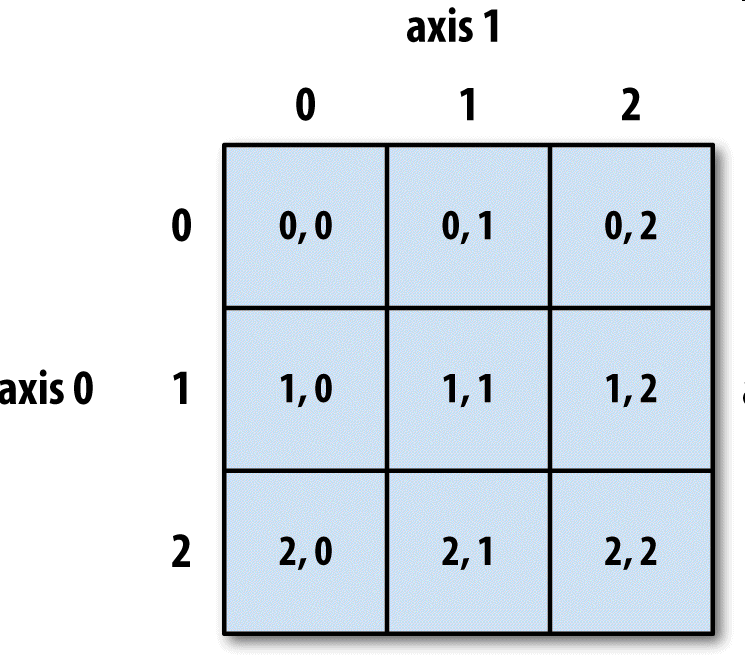In : arr3d = np.array([[[1, 2, 3], [4, 5, 6]], [[7, 8, 9], [10, 11, 12]]])

In : arr3d
Out:
array([[[ 1,  2,  3],
[ 4,  5,  6]],
[[ 7,  8,  9],
[10, 11, 12]]])


arr3d是一个2×3数组：

In : arr3d
Out:
array([[1, 2, 3],
[4, 5, 6]])


In : old_values = arr3d.copy()

In : arr3d = 42

In : arr3d
Out:
array([[[42, 42, 42],
[42, 42, 42]],
[[ 7,  8,  9],
[10, 11, 12]]])

In : arr3d = old_values

In : arr3d
Out:
array([[[ 1,  2,  3],
[ 4,  5,  6]],
[[ 7,  8,  9],
[10, 11, 12]]])


In : arr3d[1, 0]
Out: array([7, 8, 9])


In : x = arr3d

In : x
Out:
array([[ 7,  8,  9],
[10, 11, 12]])

In : x
Out: array([7, 8, 9])


## 切片索引

ndarray的切片语法跟Python列表这样的一维对象差不多：

In : arr
Out: array([ 0,  1,  2,  3,  4, 64, 64, 64,  8,  9])

In : arr[1:6]
Out: array([ 1,  2,  3,  4, 64])


In : arr2d
Out:
array([[1, 2, 3],
[4, 5, 6],
[7, 8, 9]])

In : arr2d[:2]
Out:
array([[1, 2, 3],
[4, 5, 6]])


In : arr2d[:2, 1:]
Out:
array([[2, 3],
[5, 6]])


In : arr2d[1, :2]
Out: array([4, 5])


In : arr2d[:2, 2]
Out: array([3, 6])


In : arr2d[:, :1]
Out:
array([,
,
])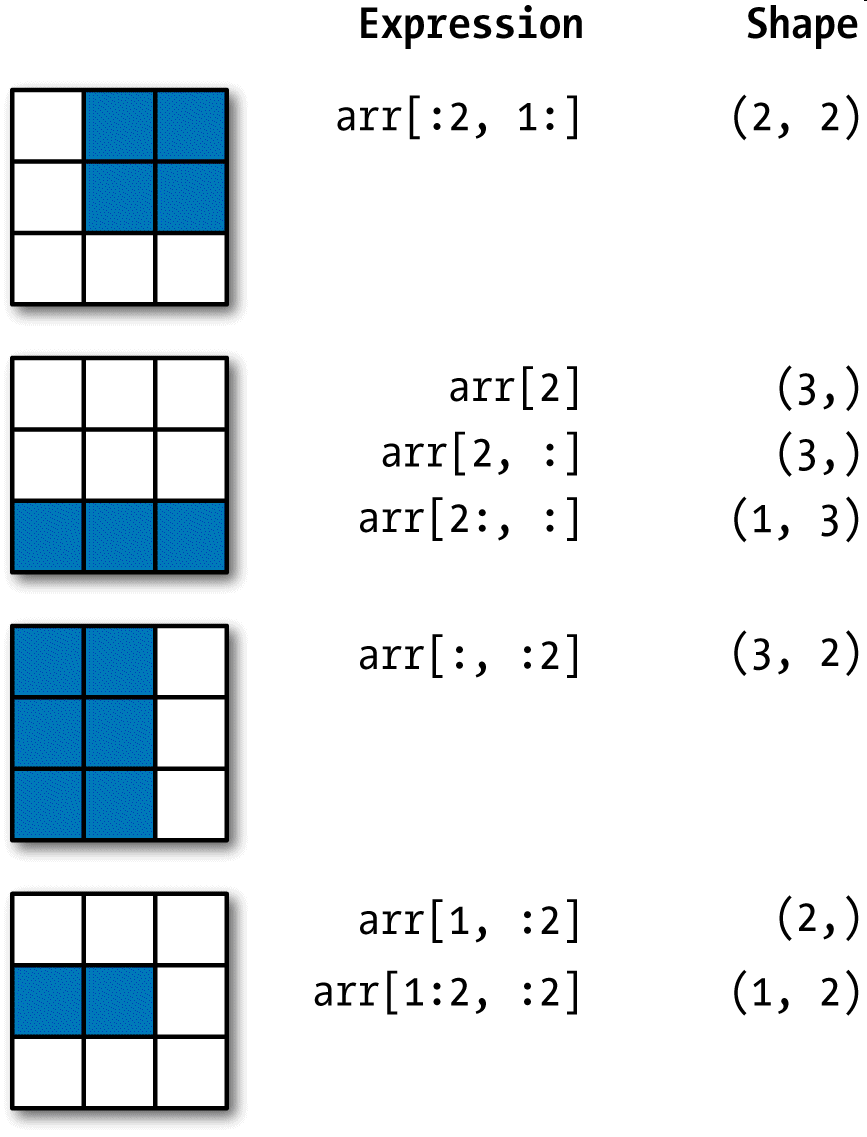In : arr2d[:2, 1:] = 0

In : arr2d
Out:
array([[1, 0, 0],
[4, 0, 0],
[7, 8, 9]])


## 布尔型索引

In : names = np.array(['Bob', 'Joe', 'Will', 'Bob', 'Will', 'Joe', 'Joe'])

In : data = np.random.randn(7, 4)

In : names
Out:
array(['Bob', 'Joe', 'Will', 'Bob', 'Will', 'Joe', 'Joe'],
dtype='<U4')

In : data
Out:
array([[ 0.0929,  0.2817,  0.769 ,  1.2464],
[ 1.0072, -1.2962,  0.275 ,  0.2289],
[ 1.3529,  0.8864, -2.0016, -0.3718],
[ 1.669 , -0.4386, -0.5397,  0.477 ],
[ 3.2489, -1.0212, -0.5771,  0.1241],
[ 0.3026,  0.5238,  0.0009,  1.3438],
[-0.7135, -0.8312, -2.3702, -1.8608]])


In : names == 'Bob'
Out: array([ True, False, False,  True, False, False, False], dtype=bool)


In : data[names == 'Bob']
Out:
array([[ 0.0929,  0.2817,  0.769 ,  1.2464],
[ 1.669 , -0.4386, -0.5397,  0.477 ]])


In : data[names == 'Bob']
Out:
array([[ 0.0929,  0.2817,  0.769 ,  1.2464],
[ 1.669 , -0.4386, -0.5397,  0.477 ]])


In : data[names == 'Bob', 2:]
Out:
array([[ 0.769 ,  1.2464],
[-0.5397,  0.477 ]])

In : data[names == 'Bob', 3]
Out: array([ 1.2464,  0.477 ])


In : names != 'Bob'
Out: array([False,  True,  True, False,  True,  True,  True], dtype=bool)

In : data[~(names == 'Bob')]
Out:
array([[ 1.0072, -1.2962,  0.275 ,  0.2289],
[ 1.3529,  0.8864, -2.0016, -0.3718],
[ 3.2489, -1.0212, -0.5771,  0.1241],
[ 0.3026,  0.5238,  0.0009,  1.3438],
[-0.7135, -0.8312, -2.3702, -1.8608]])


~操作符用来反转条件很好用：

In : cond = names == 'Bob'

In : data[~cond]
Out:
array([[ 1.0072, -1.2962,  0.275 ,  0.2289],
[ 1.3529,  0.8864, -2.0016, -0.3718],
[ 3.2489, -1.0212, -0.5771,  0.1241],
[ 0.3026,  0.5238,  0.0009,  1.3438],
[-0.7135, -0.8312, -2.3702, -1.8608]])


In : mask = (names == 'Bob') | (names == 'Will')

Out: array([ True, False,  True,  True,  True, False, False], dtype=bool)

Out:
array([[ 0.0929,  0.2817,  0.769 ,  1.2464],
[ 1.3529,  0.8864, -2.0016, -0.3718],
[ 1.669 , -0.4386, -0.5397,  0.477 ],
[ 3.2489, -1.0212, -0.5771,  0.1241]])


In : data[data < 0] = 0

In : data
Out:
array([[ 0.0929,  0.2817,  0.769 ,  1.2464],
[ 1.0072,  0.    ,  0.275 ,  0.2289],
[ 1.3529,  0.8864,  0.    ,  0.    ],
[ 1.669 ,  0.    ,  0.    ,  0.477 ],
[ 3.2489,  0.    ,  0.    ,  0.1241],
[ 0.3026,  0.5238,  0.0009,  1.3438],
[ 0.    ,  0.    ,  0.    ,  0.    ]])


In : data[names != 'Joe'] = 7

In : data
Out:
array([[ 7.    ,  7.    ,  7.    ,  7.    ],
[ 1.0072,  0.    ,  0.275 ,  0.2289],
[ 7.    ,  7.    ,  7.    ,  7.    ],
[ 7.    ,  7.    ,  7.    ,  7.    ],
[ 7.    ,  7.    ,  7.    ,  7.    ],
[ 0.3026,  0.5238,  0.0009,  1.3438],
[ 0.    ,  0.    ,  0.    ,  0.    ]])


## 花式索引

In : arr = np.empty((8, 4))

In : for i in range(8):
.....:     arr[i] = i

In : arr
Out:
array([[ 0.,  0.,  0.,  0.],
[ 1.,  1.,  1.,  1.],
[ 2.,  2.,  2.,  2.],
[ 3.,  3.,  3.,  3.],
[ 4.,  4.,  4.,  4.],
[ 5.,  5.,  5.,  5.],
[ 6.,  6.,  6.,  6.],
[ 7.,  7.,  7.,  7.]])


In : arr[[4, 3, 0, 6]]
Out:
array([[ 4.,  4.,  4.,  4.],
[ 3.,  3.,  3.,  3.],
[ 0.,  0.,  0.,  0.],
[ 6.,  6.,  6.,  6.]])


In : arr[[-3, -5, -7]]
Out:
array([[ 5.,  5.,  5.,  5.],
[ 3.,  3.,  3.,  3.],
[ 1.,  1.,  1.,  1.]])


In : arr = np.arange(32).reshape((8, 4))

In : arr
Out:
array([[ 0,  1,  2,  3],
[ 4,  5,  6,  7],
[ 8,  9, 10, 11],
[12, 13, 14, 15],
[16, 17, 18, 19],
[20, 21, 22, 23],
[24, 25, 26, 27],
[28, 29, 30, 31]])

In : arr[[1, 5, 7, 2], [0, 3, 1, 2]]
Out: array([ 4, 23, 29, 10])


In : arr[[1, 5, 7, 2]][:, [0, 3, 1, 2]]
Out:
array([[ 4,  7,  5,  6],
[20, 23, 21, 22],
[28, 31, 29, 30],
[ 8, 11,  9, 10]])


## 数组转置和轴对换

In : arr = np.arange(15).reshape((3, 5))

In : arr
Out:
array([[ 0,  1,  2,  3,  4],
[ 5,  6,  7,  8,  9],
[10, 11, 12, 13, 14]])

In : arr.T
Out:
array([[ 0,  5, 10],
[ 1,  6, 11],
[ 2,  7, 12],
[ 3,  8, 13],
[ 4,  9, 14]])


In : arr = np.random.randn(6, 3)

In : arr
Out:
array([[-0.8608,  0.5601, -1.2659],
[ 0.1198, -1.0635,  0.3329],
[-2.3594, -0.1995, -1.542 ],
[-0.9707, -1.307 ,  0.2863],
[ 0.378 , -0.7539,  0.3313],
[ 1.3497,  0.0699,  0.2467]])

In : np.dot(arr.T, arr)
Out:
array([[ 9.2291,  0.9394,  4.948 ],
[ 0.9394,  3.7662, -1.3622],
[ 4.948 , -1.3622,  4.3437]])


In : arr = np.arange(16).reshape((2, 2, 4))

In : arr
Out:
array([[[ 0,  1,  2,  3],
[ 4,  5,  6,  7]],
[[ 8,  9, 10, 11],
[12, 13, 14, 15]]])

In : arr.transpose((1, 0, 2))
Out:
array([[[ 0,  1,  2,  3],
[ 8,  9, 10, 11]],
[[ 4,  5,  6,  7],
[12, 13, 14, 15]]])


In : arr
Out:
array([[[ 0,  1,  2,  3],
[ 4,  5,  6,  7]],
[[ 8,  9, 10, 11],
[12, 13, 14, 15]]])

In : arr.swapaxes(1, 2)
Out:
array([[[ 0,  4],
[ 1,  5],
[ 2,  6],
[ 3,  7]],
[[ 8, 12],
[ 9, 13],
[10, 14],
[11, 15]]])


swapaxes也是返回源数据的视图（不会进行任何复制操作）。

# 4.2 通用函数：快速的元素级数组函数

In : arr = np.arange(10)

In : arr
Out: array([0, 1, 2, 3, 4, 5, 6, 7, 8, 9])

In : np.sqrt(arr)
Out:
array([ 0.    ,  1.    ,  1.4142,  1.7321,  2.    ,  2.2361,  2.4495,
2.6458,  2.8284,  3.    ])

In : np.exp(arr)
Out:
array([    1.    ,     2.7183,     7.3891,    20.0855,    54.5982,
148.4132,   403.4288,  1096.6332,  2980.958 ,  8103.0839])


In : x = np.random.randn(8)

In : y = np.random.randn(8)

In : x
Out:
array([-0.0119,  1.0048,  1.3272, -0.9193, -1.5491,  0.0222,  0.7584,
-0.6605])

In : y
Out:
array([ 0.8626, -0.01  ,  0.05  ,  0.6702,  0.853 , -0.9559, -0.0235,
-2.3042])

In : np.maximum(x, y)
Out:
array([ 0.8626,  1.0048,  1.3272,  0.6702,  0.853 ,  0.0222,  0.7584,
-0.6605])


In : arr = np.random.randn(7) * 5

In : arr
Out: array([-3.2623, -6.0915, -6.663 ,  5.3731,  3.6182,  3.45  ,  5.0077])

In : remainder, whole_part = np.modf(arr)

In : remainder
Out: array([-0.2623, -0.0915, -0.663 ,  0.3731,
0.6182,  0.45  ,  0.0077])

In : whole_part
Out: array([-3., -6., -6.,  5.,  3.,  3.,  5.])


Ufuncs可以接受一个out可选参数，这样就能在数组原地进行操作：

In : arr
Out: array([-3.2623, -6.0915, -6.663 ,  5.3731,  3.6182,  3.45  ,  5.0077])

In : np.sqrt(arr)
Out: array([    nan,     nan,     nan,  2.318 ,  1.9022,  1.8574,  2.2378])

In : np.sqrt(arr, arr)
Out: array([    nan,     nan,     nan,  2.318 ,  1.9022,  1.8574,  2.2378])

In : arr
Out: array([    nan,     nan,     nan,  2.318 ,  1.9022,  1.8574,  2.2378])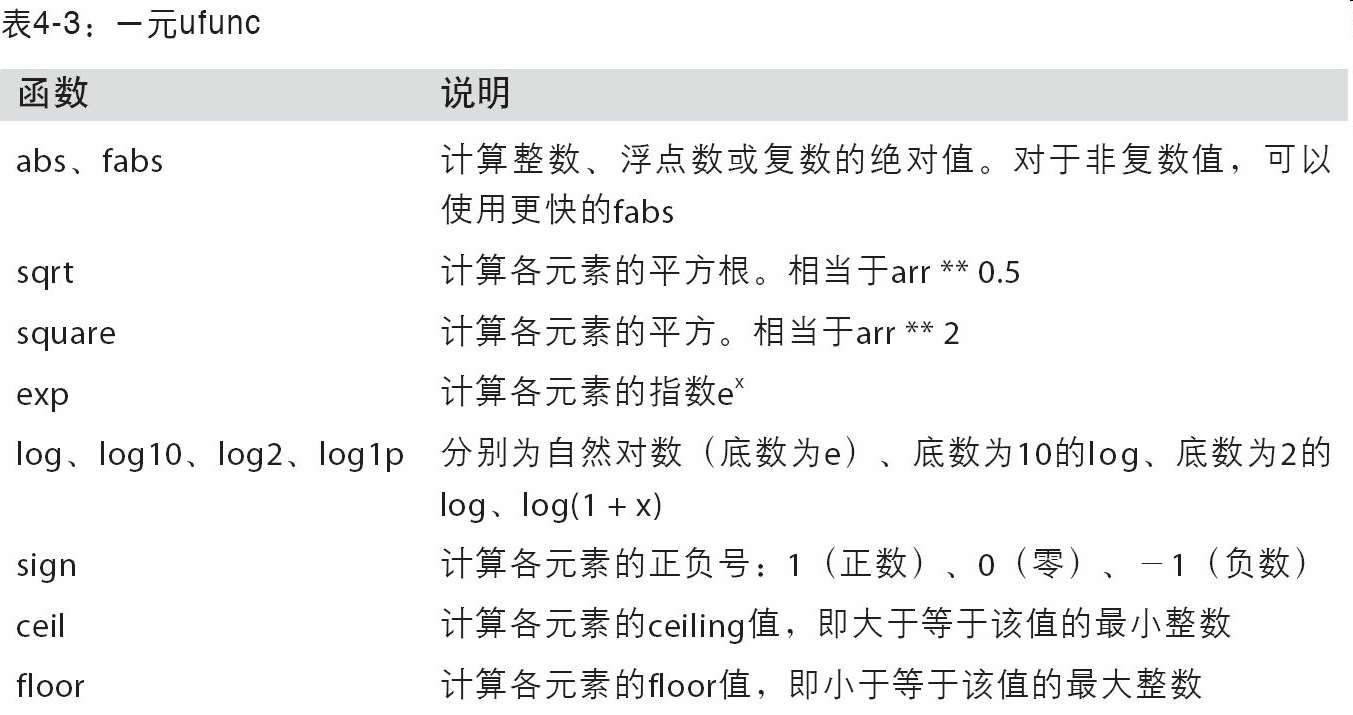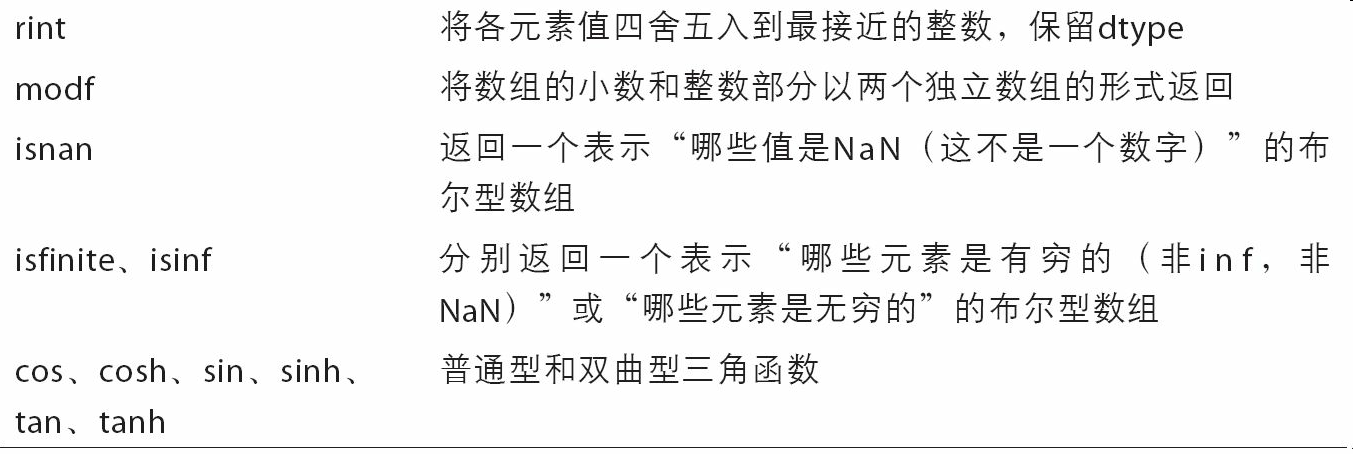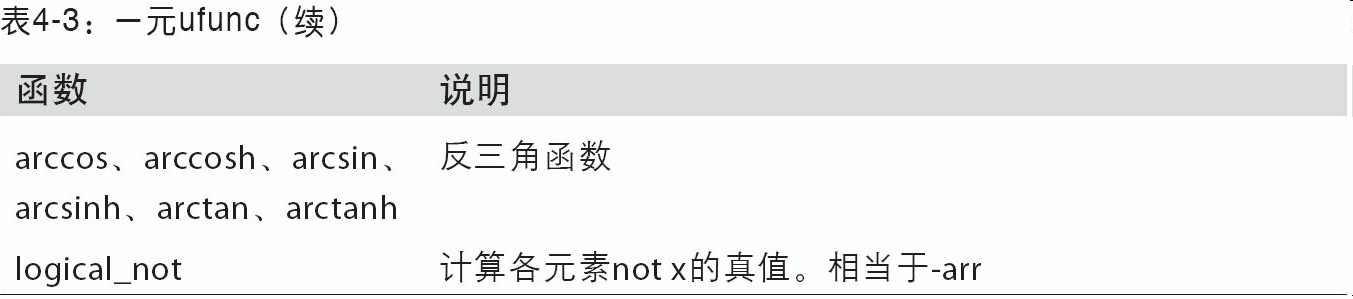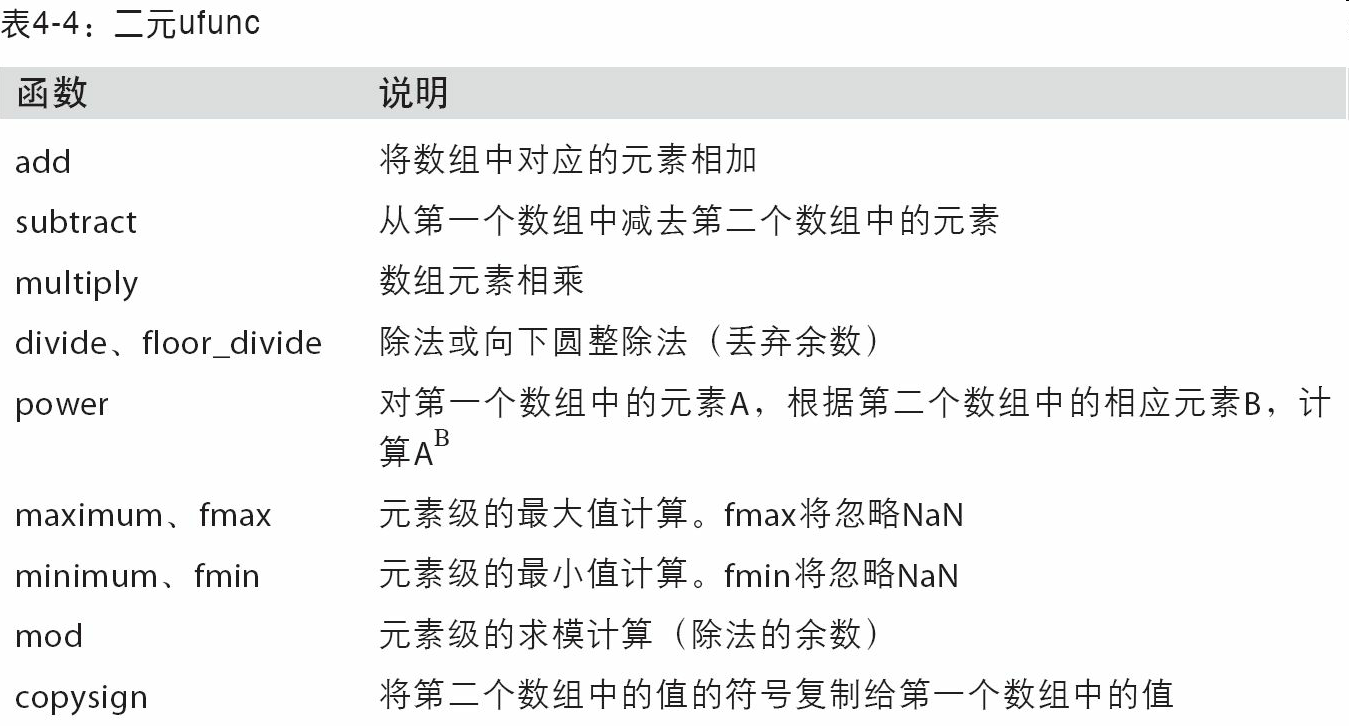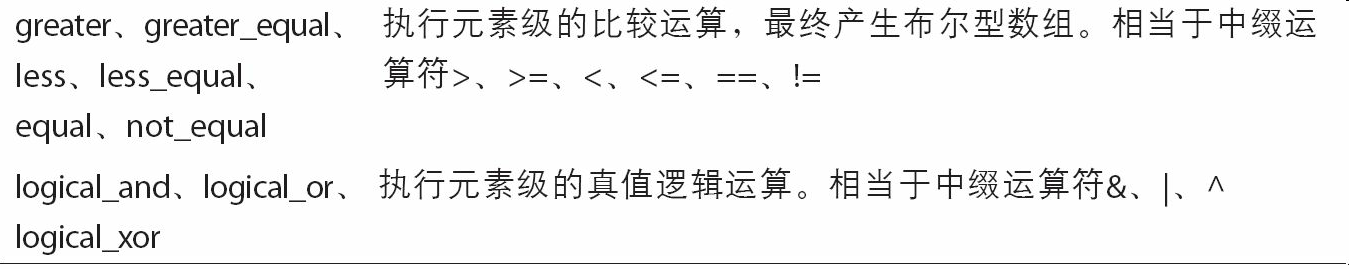# 4.3 利用数组进行数据处理

NumPy数组使你可以将许多种数据处理任务表述为简洁的数组表达式（否则需要编写循环）。用数组表达式代替循环的做法，通常被称为矢量化。一般来说，矢量化数组运算要比等价的纯Python方式快上一两个数量级（甚至更多），尤其是各种数值计算。在后面内容中（见附录A）我将介绍广播，这是一种针对矢量化计算的强大手段。

In : points = np.arange(-5, 5, 0.01) # 1000 equally spaced points

In : xs, ys = np.meshgrid(points, points)
In : ys
Out:
array([[-5.  , -5.  , -5.  , ..., -5.  , -5.  , -5.  ],
[-4.99, -4.99, -4.99, ..., -4.99, -4.99, -4.99],
[-4.98, -4.98, -4.98, ..., -4.98, -4.98, -4.98],
...,
[ 4.97,  4.97,  4.97, ...,  4.97,  4.97,  4.97],
[ 4.98,  4.98,  4.98, ...,  4.98,  4.98,  4.98],
[ 4.99,  4.99,  4.99, ...,  4.99,  4.99,  4.99]])


In : z = np.sqrt(xs ** 2 + ys ** 2)

In : z
Out:
array([[ 7.0711,  7.064 ,  7.0569, ...,  7.0499,  7.0569,  7.064 ],
[ 7.064 ,  7.0569,  7.0499, ...,  7.0428,  7.0499,  7.0569],
[ 7.0569,  7.0499,  7.0428, ...,  7.0357,  7.0428, 7.0499],
...,
[ 7.0499,  7.0428,  7.0357, ...,  7.0286,  7.0357,  7.0428],
[ 7.0569,  7.0499,  7.0428, ...,  7.0357,  7.0428,  7.0499],
[ 7.064 ,  7.0569,  7.0499, ...,  7.0428,  7.0499,  7.0569]])


In : import matplotlib.pyplot as plt

In : plt.imshow(z, cmap=plt.cm.gray); plt.colorbar()
Out: <matplotlib.colorbar.Colorbar at 0x7f715e3fa630>

In : plt.title("Image plot of $\sqrt{x^2 + y^2}$ for a grid of values")
Out: <matplotlib.text.Text at 0x7f715d2de748>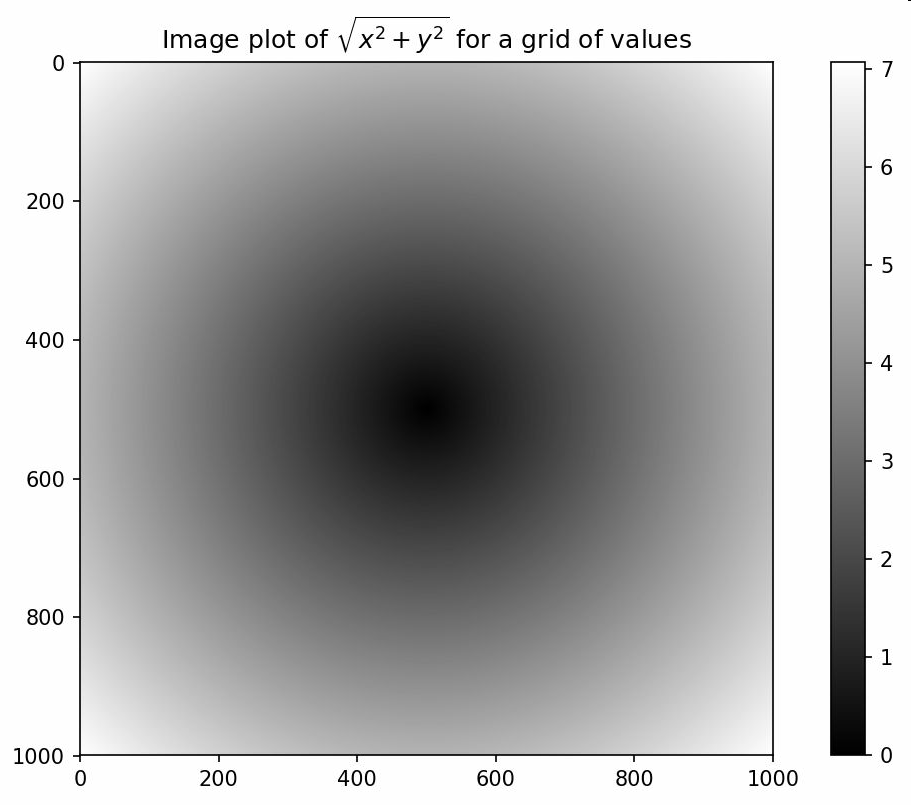## 将条件逻辑表述为数组运算

numpy.where函数是三元表达式x if condition else y的矢量化版本。假设我们有一个布尔数组和两个值数组：

In : xarr = np.array([1.1, 1.2, 1.3, 1.4, 1.5])

In : yarr = np.array([2.1, 2.2, 2.3, 2.4, 2.5])

In : cond = np.array([True, False, True, True, False])


In : result = [(x if c else y)
.....:           for x, y, c in zip(xarr, yarr, cond)]

In : result
Out: [1.1000000000000001, 2.2000000000000002, 1.3, 1.3999999999999999, 2.5]


In : result = np.where(cond, xarr, yarr)

In : result
Out: array([ 1.1,  2.2,  1.3,  1.4,  2.5])


np.where的第二个和第三个参数不必是数组，它们都可以是标量值。在数据分析工作中，where通常用于根据另一个数组而产生一个新的数组。假设有一个由随机数据组成的矩阵，你希望将所有正值替换为2，将所有负值替换为－2。若利用np.where，则会非常简单：

In : arr = np.random.randn(4, 4)

In : arr
Out:
array([[-0.5031, -0.6223, -0.9212, -0.7262],
[ 0.2229,  0.0513, -1.1577,  0.8167],
[ 0.4336,  1.0107,  1.8249, -0.9975],
[ 0.8506, -0.1316,  0.9124,  0.1882]])

In : arr > 0
Out:
array([[False, False, False, False],
[ True,  True, False,  True],
[ True,  True,  True, False],
[ True, False,  True,  True]], dtype=bool)

In : np.where(arr > 0, 2, -2)
Out:
array([[-2, -2, -2, -2],
[ 2,  2, -2,  2],
[ 2,  2,  2, -2],
[ 2, -2,  2,  2]])


In : np.where(arr > 0, 2, arr) # set only positive values to 2
Out:
array([[-0.5031, -0.6223, -0.9212, -0.7262],
[ 2.    ,  2.    , -1.1577,  2.    ],
[ 2.    ,  2.    ,  2.    , -0.9975],
[ 2.    , -0.1316,  2.    ,  2.    ]])


## 数学和统计方法

In : arr = np.random.randn(5, 4)

In : arr
Out:
array([[ 2.1695, -0.1149,  2.0037,  0.0296],
[ 0.7953,  0.1181, -0.7485,  0.585 ],
[ 0.1527, -1.5657, -0.5625, -0.0327],
[-0.929 , -0.4826, -0.0363,  1.0954],
[ 0.9809, -0.5895,  1.5817, -0.5287]])

In : arr.mean()
Out: 0.19607051119998253

In : np.mean(arr)
Out: 0.19607051119998253

In : arr.sum()
Out: 3.9214102239996507


mean和sum这类的函数可以接受一个axis选项参数，用于计算该轴向上的统计值，最终结果是一个少一维的数组：

In : arr.mean(axis=1)
Out: array([ 1.022 ,  0.1875, -0.502 , -0.0881,  0.3611])

In : arr.sum(axis=0)
Out: array([ 3.1693, -2.6345,  2.2381,  1.1486])


In : arr = np.array([0, 1, 2, 3, 4, 5, 6, 7])

In : arr.cumsum()
Out: array([ 0,  1,  3,  6, 10, 15, 21, 28])


In : arr = np.array([[0, 1, 2], [3, 4, 5], [6, 7, 8]])

In : arr
Out:
array([[0, 1, 2],
[3, 4, 5],
[6, 7, 8]])

In : arr.cumsum(axis=0)
Out:
array([[ 0,  1,  2],
[ 3,  5,  7],
[ 9, 12, 15]])

In : arr.cumprod(axis=1)
Out:
array([[  0,   0,   0],
[  3,  12,  60],
[  6,  42, 336]])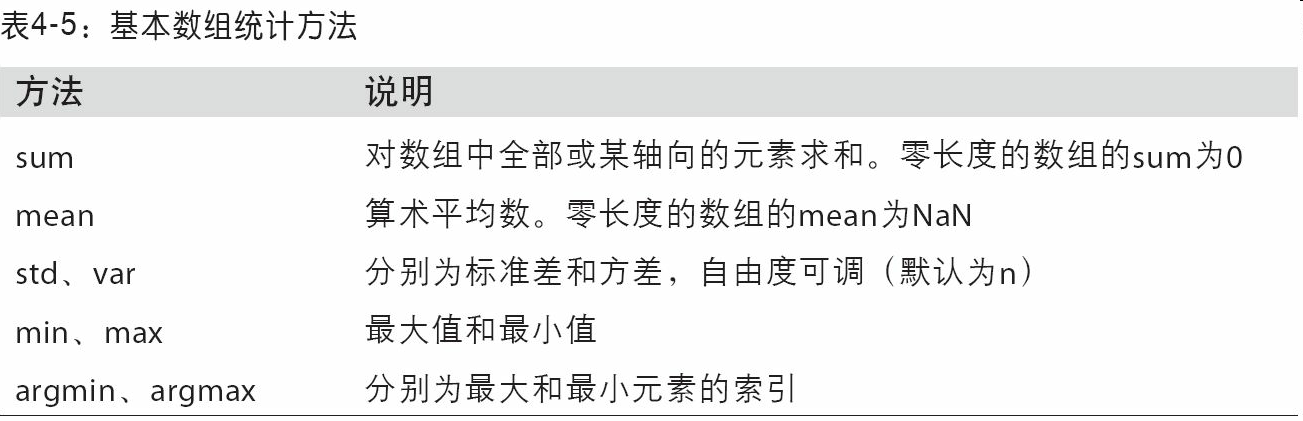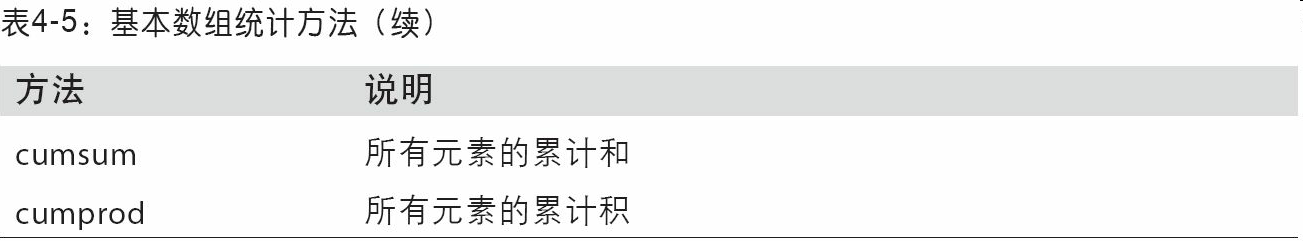## 用于布尔型数组的方法

In : arr = np.random.randn(100)

In : (arr > 0).sum() # Number of positive values
Out: 42


In : bools = np.array([False, False, True, False])

In : bools.any()
Out: True

In : bools.all()
Out: False


## 排序

In : arr = np.random.randn(6)

In : arr
Out: array([ 0.6095, -0.4938,  1.24  , -0.1357,  1.43  , -0.8469])

In : arr.sort()

In : arr
Out: array([-0.8469, -0.4938, -0.1357,  0.6095,  1.24  ,  1.43  ])


In : arr = np.random.randn(5, 3)

In : arr
Out:
array([[ 0.6033,  1.2636, -0.2555],
[-0.4457,  0.4684, -0.9616],
[-1.8245,  0.6254,  1.0229],
[ 1.1074,  0.0909, -0.3501],
[ 0.218 , -0.8948, -1.7415]])

In : arr.sort(1)

In : arr
Out:
array([[-0.2555,  0.6033,  1.2636],
[-0.9616, -0.4457,  0.4684],
[-1.8245,  0.6254,  1.0229],
[-0.3501,  0.0909,  1.1074],
[-1.7415, -0.8948,  0.218 ]])


In : large_arr = np.random.randn(1000)

In : large_arr.sort()

In : large_arr[int(0.05 * len(large_arr))] # 5% quantile
Out: -1.5311513550102103


## 唯一化以及其它的集合逻辑

NumPy提供了一些针对一维ndarray的基本集合运算。最常用的可能要数np.unique了，它用于找出数组中的唯一值并返回已排序的结果：

In : names = np.array(['Bob', 'Joe', 'Will', 'Bob', 'Will', 'Joe', 'Joe'])

In : np.unique(names)
Out:
array(['Bob', 'Joe', 'Will'],
dtype='<U4')

In : ints = np.array([3, 3, 3, 2, 2, 1, 1, 4, 4])

In : np.unique(ints)
Out: array([1, 2, 3, 4])


In : sorted(set(names))
Out: ['Bob', 'Joe', 'Will']


In : values = np.array([6, 0, 0, 3, 2, 5, 6])

In : np.in1d(values, [2, 3, 6])
Out: array([ True, False, False,  True,  True, False,  True], dtype=bool)


NumPy中的集合函数请参见表4-6。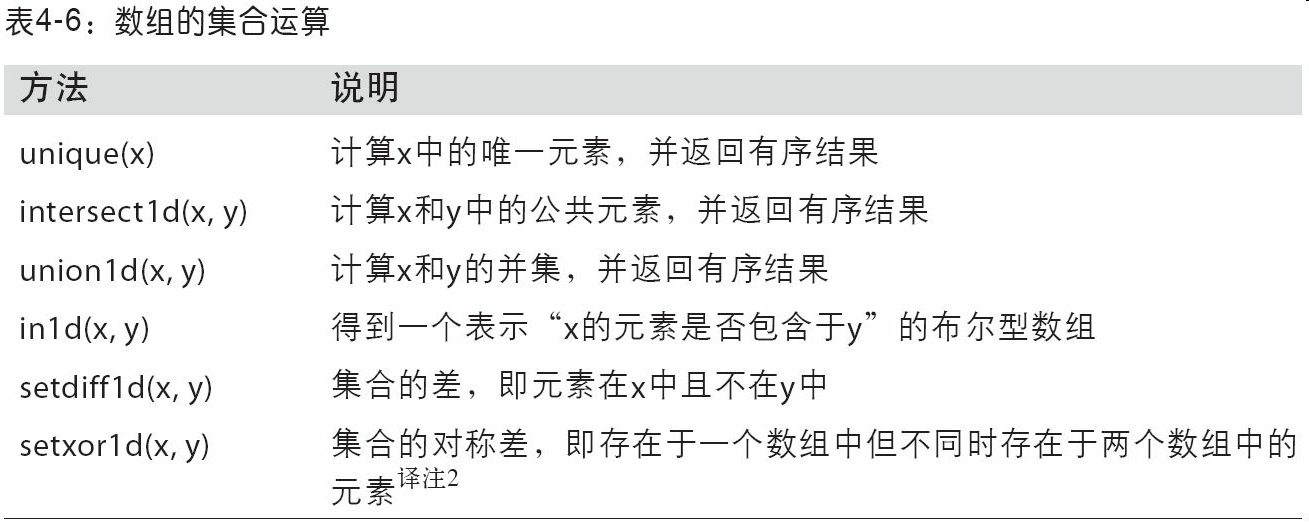# 4.4 用于数组的文件输入输出

NumPy能够读写磁盘上的文本数据或二进制数据。这一小节只讨论NumPy的内置二进制格式，因为更多的用户会使用pandas或其它工具加载文本或表格数据（见第6章）。

In : arr = np.arange(10)

In : np.save('some_array', arr)


In : np.load('some_array.npy')
Out: array([0, 1, 2, 3, 4, 5, 6, 7, 8, 9])


In : np.savez('array_archive.npz', a=arr, b=arr)


In : arch = np.load('array_archive.npz')

In : arch['b']
Out: array([0, 1, 2, 3, 4, 5, 6, 7, 8, 9])


In : np.savez_compressed('arrays_compressed.npz', a=arr, b=arr)


# 4.5 线性代数

In : x = np.array([[1., 2., 3.], [4., 5., 6.]])

In : y = np.array([[6., 23.], [-1, 7], [8, 9]])

In : x
Out:
array([[ 1.,  2.,  3.],
[ 4.,  5.,  6.]])

In : y
Out:
array([[  6.,  23.],
[ -1.,   7.],
[  8.,   9.]])

In : x.dot(y)
Out:
array([[  28.,   64.],
[  67.,  181.]])


x.dot(y)等价于np.dot(x, y)：

In : np.dot(x, y)
Out:
array([[  28.,   64.],
[  67.,  181.]])


In : np.dot(x, np.ones(3))
Out: array([  6.,  15.])


@符（类似Python 3.5）也可以用作中缀运算符，进行矩阵乘法：

In : x @ np.ones(3)
Out: array([  6.,  15.])


numpy.linalg中有一组标准的矩阵分解运算以及诸如求逆和行列式之类的东西。它们跟MATLAB和R等语言所使用的是相同的行业标准线性代数库，如BLAS、LAPACK、Intel MKL（Math Kernel Library，可能有，取决于你的NumPy版本）等：

In : from numpy.linalg import inv, qr

In : X = np.random.randn(5, 5)

In : mat = X.T.dot(X)

In : inv(mat)
Out:
array([[  933.1189,   871.8258, -1417.6902, -1460.4005,  1782.1391],
[  871.8258,   815.3929, -1325.9965, -1365.9242,  1666.9347],
[-1417.6902, -1325.9965,  2158.4424,  2222.0191, -2711.6822],
[-1460.4005, -1365.9242,  2222.0191,  2289.0575, -2793.422 ],
[ 1782.1391,  1666.9347, -2711.6822, -2793.422 ,  3409.5128]])

In : mat.dot(inv(mat))
Out:
array([[ 1.,  0., -0., -0., -0.],
[-0.,  1.,  0.,  0.,  0.],
[ 0.,  0.,  1.,  0.,  0.],
[-0.,  0.,  0.,  1., -0.],
[-0.,  0.,  0.,  0.,  1.]])

In : q, r = qr(mat)

In : r
Out:
array([[-1.6914,  4.38  ,  0.1757,  0.4075, -0.7838],
[ 0.    , -2.6436,  0.1939, -3.072 , -1.0702],
[ 0.    ,  0.    , -0.8138,  1.5414,  0.6155],
[ 0.    ,  0.    ,  0.    , -2.6445, -2.1669],
[ 0.    ,  0.    ,  0.    ,  0.    ,  0.0002]])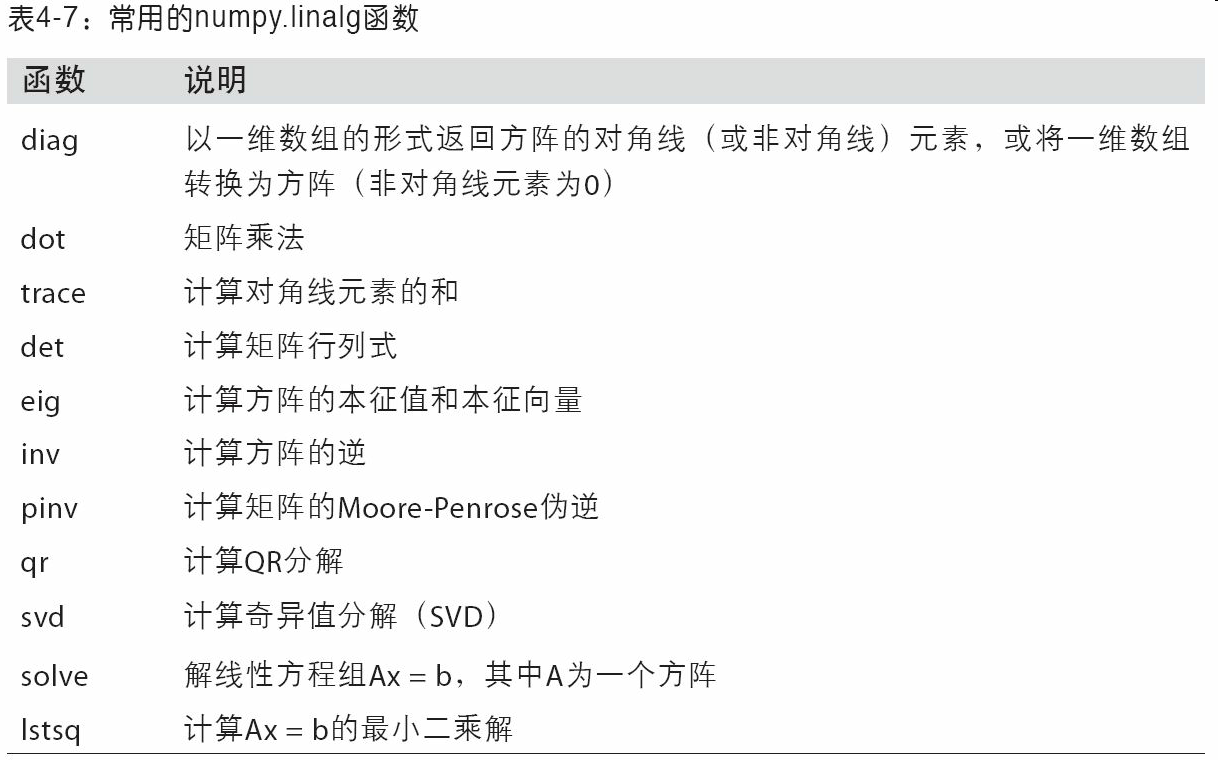# 4.6 伪随机数生成

numpy.random模块对Python内置的random进行了补充，增加了一些用于高效生成多种概率分布的样本值的函数。例如，你可以用normal来得到一个标准正态分布的4×4样本数组：

In : samples = np.random.normal(size=(4, 4))

In : samples
Out:
array([[ 0.5732,  0.1933,  0.4429,  1.2796],
[ 0.575 ,  0.4339, -0.7658, -1.237 ],
[-0.5367,  1.8545, -0.92  , -0.1082],
[ 0.1525,  0.9435, -1.0953, -0.144 ]])


In : from random import normalvariate

In : N = 1000000

In : %timeit samples = [normalvariate(0, 1) for _ in range(N)]
1.77 s +- 126 ms per loop (mean +- std. dev. of 7 runs, 1 loop each)

In : %timeit np.random.normal(size=N)
61.7 ms +- 1.32 ms per loop (mean +- std. dev. of 7 runs, 10 loops each)


In : np.random.seed(1234)


numpy.random的数据生成函数使用了全局的随机种子。要避免全局状态，你可以使用numpy.random.RandomState，创建一个与其它隔离的随机数生成器：

In : rng = np.random.RandomState(1234)

In : rng.randn(10)
Out:
array([ 0.4714, -1.191 ,  1.4327, -0.3127, -0.7206,  0.8872,  0.8596,
-0.6365,  0.0157, -2.2427])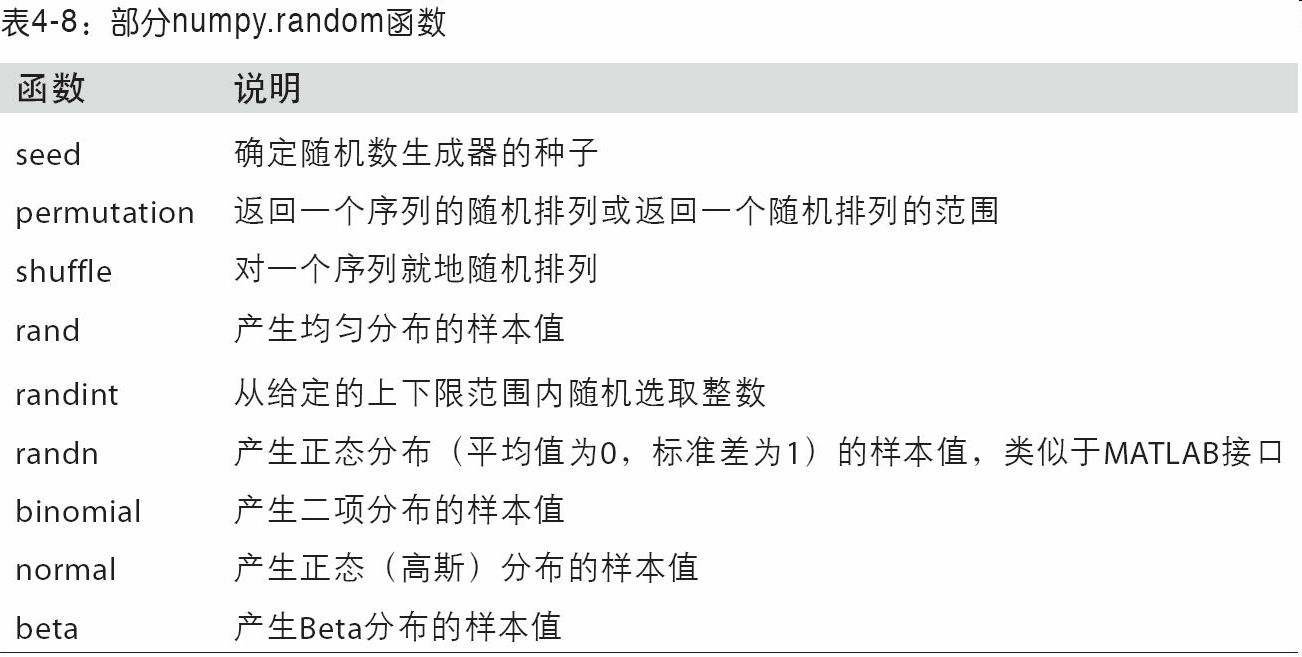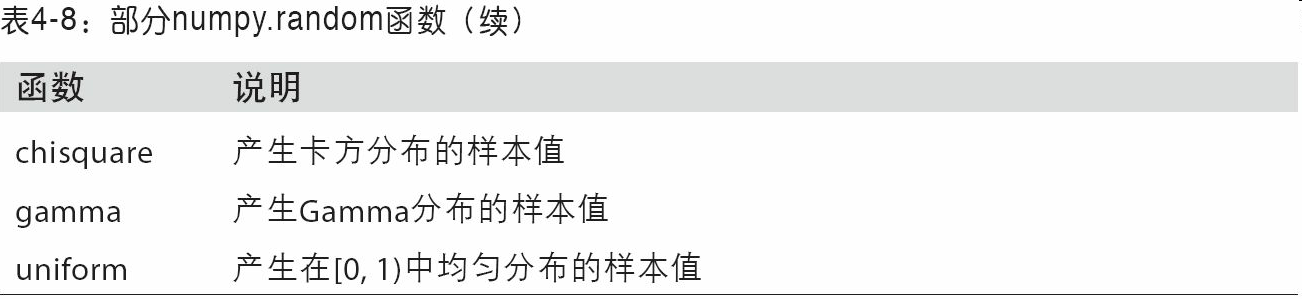# 4.7 示例：随机漫步

In : import random
.....: position = 0
.....: walk = [position]
.....: steps = 1000
.....: for i in range(steps):
.....:     step = 1 if random.randint(0, 1) else -1
.....:     position += step
.....:     walk.append(position)
.....:


In : plt.plot(walk[:100])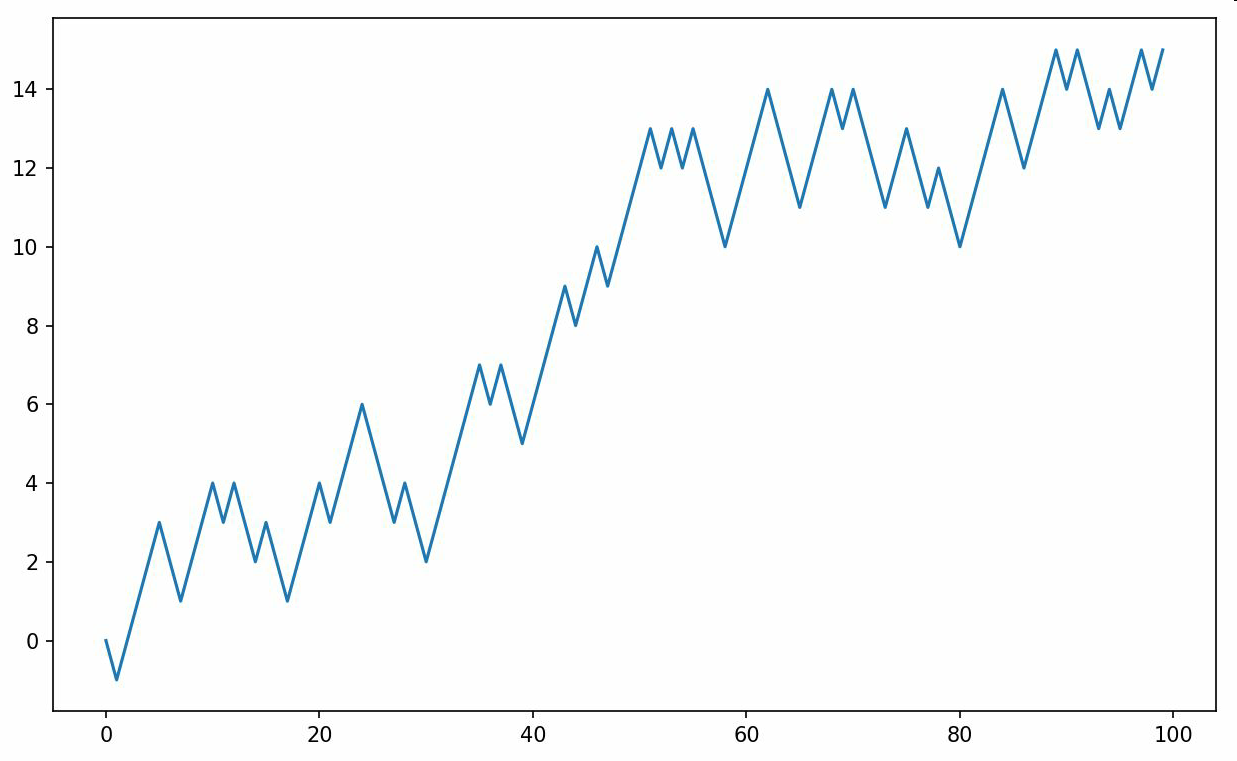In : nsteps = 1000

In : draws = np.random.randint(0, 2, size=nsteps)

In : steps = np.where(draws > 0, 1, -1)

In : walk = steps.cumsum()


In : walk.min()
Out: -3

In : walk.max()
Out: 31


In : (np.abs(walk) >= 10).argmax()
Out: 37


## 一次模拟多个随机漫步

In : nwalks = 5000

In : nsteps = 1000

In : draws = np.random.randint(0, 2, size=(nwalks, nsteps)) # 0 or 1

In : steps = np.where(draws > 0, 1, -1)

In : walks = steps.cumsum(1)

In : walks
Out:
array([[  1,   0,   1, ...,   8,   7,   8],
[  1,   0,  -1, ...,  34,  33,  32],
[  1,   0,  -1, ...,   4,   5,   4],
...,
[  1,   2,   1, ...,  24,  25,  26],
[  1,   2,   3, ...,  14,  13,  14],
[ -1,  -2,  -3, ..., -24, -23, -22]])


In : walks.max()
Out: 138

In : walks.min()
Out: -133


In : hits30 = (np.abs(walks) >= 30).any(1)

In : hits30
Out: array([False,  True, False, ..., False,  True, False], dtype=bool)

In : hits30.sum() # Number that hit 30 or -30
Out: 3410


In : crossing_times = (np.abs(walks[hits30]) >= 30).argmax(1)

In : crossing_times.mean()
Out: 498.88973607038122


In : steps = np.random.normal(loc=0, scale=0.25,
.....:                          size=(nwalks, nsteps))


seancheney
+ 关注